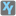• # Confusion about the task

## Question related to missionReverse Engineer

I think it's impossible to guess out the specific answer if there is no limit on the target expression.(Or limits in the description is not enough. According to other discussions, length of expression, max amounts of variables, etc. may be some supplements.)

The task is like to fit a series of nodes with a curved surface. Consider the fraction denotion of the result, it's convenient to regard the final answer as a quotient of two polynomials. By carefully select the maximum power of x, y and cross-term, we can construct full-rank equations set with same amount of parameters and equations both on numeritor and denominator, which means that, we can solve for an "right" answer with no matter how much guesses and the answer will fit for each guess perfectly but hardly be just the target answer.